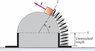# Elastic Force over Semicircle

• Peach
I think you're right, the system might interpret arcsin(theta) as arcsin.In summary, the homework statement is an online assignment that asks you to calculate the work done by a force on a block that is moved slowly through a semicircle.f

## Homework Statement

A variable force F_vec is maintained tangent to a frictionless, semicircular surface View Figure . By slowly varying the force, a block with weight w is moved through the angla theta, and the spring to which it is attached is stretched from position 1 to position 2. The spring has negligible mass and force constant k. The end of the spring moves in an arc of radius a.

Calculate the work done by the force F_vec.

## Homework Equations

K_1 + U_1 + Wother = K_2 + U_2

## The Attempt at a Solution

I'm not sure if I'm getting this correctly. Besides the elastic force, there's also the varying force correct? So that should Wother?

This is my equations so far:

K_1 = 0
U_1 = 0

K_2 = 1/2mv^2
U_2 = 1/2k(a*theta)^2

But I'm stuck on finding the velocity for K_2 in terms of a and theta. Any help is greatly appreciated, thanks. ... Assuming I'm correct so far (which I sort of doubt).

#### Attachments

•yf_Figure_7_40.jpg
5.8 KB · Views: 748
Last edited:
Actually, when the problem says it is moved very slowly, that means you can neglect the fact that it has a tiny kinetic energy. So, K_2 should be 0. The other parts of your work look correct. :)

Last edited:
I entered that and it says it's incorrect. What else am I missing? What about the weight on top of the spring? Does that have something to do with it?

Ah, yes, if the block is being raised vertically you will need to include potential energy of mgh after it is raised. (Of course you'll need to express mgh in terms of w and theta.)

So I have to add the potential energy of the box to the potential energy of the spring (which is the elastic force right)? So mgh_box = (w*a*theta)?

You do have to add the potential energy of the box to that of the spring, but be careful how you do it. Remember that h is the height the object has been raised, not the distance it has traveled along the semicircle.

The height isn't theta, is it? Wait, shouldn't the height be the radius then? >< Sorry I'm so confused about this circle thing.

It's okay, to see how much the block has been raised, draw a line on the diagram to represent the height the block has moved:

http://img255.imageshack.us/img255/3154/yffigure740st3.jpg [Broken]

Look familiar?

Last edited by a moderator:
Got it. So the total potential energy should be:

deltaU = wasin(theta) + 1/2k(a*theta)^2

Right? Just making sure because I only have one last attempt.

That looks good to me. :)

The answer is wrong. What else could we be missing? This is so frusterating. ><

I'm not sure. I just worked the problem by integrating an infinitesimal amount of work dW = F*dx and I got the same answer.

Net force acting on the block during its movement along the semicircle (mgsin(theta) and N cancel):
$$\sum F_{net} = F - kx - wcos(\theta) = 0$$

So,

$$F = wcos(\theta) + kx$$

(Remember x = a*theta and dx = a*dtheta)

$$dW = F dx \\ = (wcos(\theta) + kx) dx \\ = (wcos(\theta) + k(a \theta)) a d\theta \\$$

$$W = \int_0^{\theta_2} w\,cos \theta \,a\,d\theta\,+\,\int_0^{\theta_2} k a \theta\,a\,d\theta \\ = w\,a\,\int_0^{\theta_2} cos \theta \,d\theta\,+\,k a^2 \int_0^{\theta_2} \theta\,d\theta \\$$
$$W = w a sin(\theta_2) + \frac{1}{2}k a^2 \theta_2^2$$

where $\theta_2$ is the final angle (the initial angle is zero).

...which should be the correct answer...?

Is the homework an online assignment where you have to fill out a textbox? Could the error be in the format you entered?

Last edited:
Yeah, it's an online hw. Erm, I just checked again the answer I entered and I didn't make a mistake entering it. I don't know what's the answer since I've maxed out my attempts already. :x I'll ask my TA if it's possible. Thanks for helping me so much already, really appreciated it.

Sure, I'd be interested in knowing the answer to this problem, so keep me posted when you find out.

Peach. The answer is correct. Check the way you entered it. Make asin(theta), apparently, is not the same as a*sin(theta). The system probably screwed you over that way.

I don't remember how I entered it, whether it was asin(theta) or a*sin(theta) but the answer that I entered is showing up as asin(theta).

I can't copy it directly but this is what it's showing up as:

(w*asin(theta)) + (1/2)*k*(a*theta)^2

is there possibly a different way to write the solution? I've thought about this problem ALOT and although the answer is right, i know I'm missing something stupid.

i had the same problem as you. i entered it with the asterisk and it was fine. i believe the system interprets "asin(theta)" as arcsin?